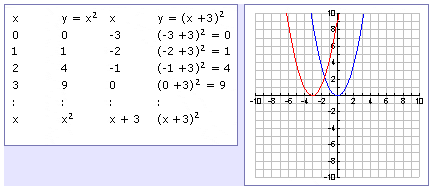Transformation
Left or Right

To understand the effects of h, compare the functions y = x2 and y = (x + 3)2. The table below shows the patterns of values.By looking at both the table of values and the graph, it appears as though h produces a horizontal shift; in this case, h = -3 shifts the parabola 3 units to the left. Expressing the equation in vertex form, y = (x + 3)2 = (x - (-3))2. In general, the graph is shifted h units to the right if h is positive, and h units to the left if h is negative.

In function notation, to shift a function left, add inside the function's argument: f(x + b) shifts f(x) b units to the left. Shifting to the right works the same way, f(x - b) shifts f(x) b units to the right.

*Note: Remember a sign on the inside of the function does the opposite as a sign on the outside of the function. This is because in order to turn f(x + b) into f(x), x = -3 which graphs to the left.